Year 10 Interactive Maths - Second Edition

## Sketch Graphs

Often we need to know the general shape and location of a graph.  In such cases, a sketch graph is drawn instead of plotting a number of points to obtain the graph.

Two points are needed to obtain a straight line graph.  It is simpler to find the points of intersection of the graph with the axes.  These points are called the x- and y- intercepts.

###### x-intercept:

The y-coordinate of any point on the x-axis is 0.  Therefore to find the x-intercept we put y = 0 in the equation and solve it for x.

###### y-intercept:

The x-coordinate of any point on the y-axis is 0.  Therefore to find the y-intercept we put x = 0 in the equation and solve it for y.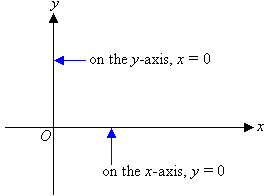#### Example 11

Sketch the graph of y = 3x + 6.

##### Solution:
y = 3x + 6

x-intercept:

######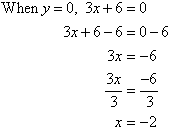y-intercept: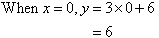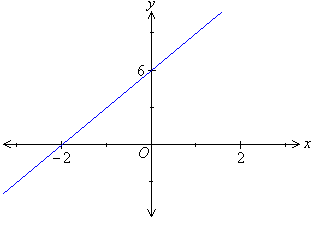###### Note:

We often represent the gradient and the y-intercept of the straight line by m and c respectively.

In the previous example: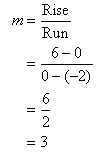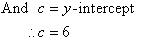From the ongoing discussion we can infer that y = 3x + 6 is a straight line with a gradient of 3 and y-intercept of 6.

In the example under consideration, the gradient of the straight line is positive.  So, the straight line slopes upward as the value of x increases.

#### Example 12

Sketch the graph of  y = 2x + 4.

##### Solution:

y = –2x + 4

x-intercept: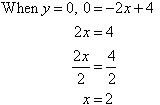y-intercept: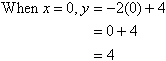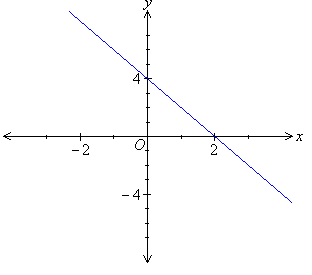###### Note: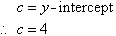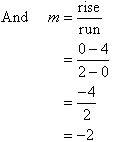From the ongoing discussion we find that the linear function y = 2x + 4 represents the equation of a straight line with a gradient of 2 and y-intercept of 4.

In the example under consideration, the gradient of the straight line is negative. So, the straight line slopes downward as the value of x increases.

#### Example 13

Sketch the graph of  y = 2x.

##### Solution:
y = 2x

x-intercept: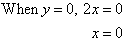y-intercept:

When x = 0, y = 0.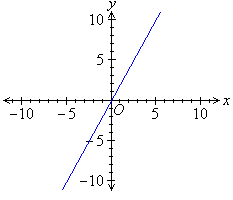As both the x- and y- intercepts are (0, 0), another point is needed.

We find when x = 5, y = 10.  So, (5, 10) is an example of another point that can be used to form the straight line graph.

###### Alternative technique:

Use the gradient-intercept method: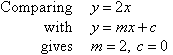So, the straight line passes through (0, 0).  Use this point to draw a line of slope 2 (i.e. go across 3 units and up 6 units).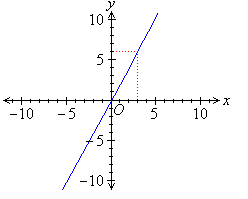###### Note:

It is simpler to find the run and rise if we start from the y-intercept.

#### Example 14

Sketch the graph of 7y – 5x = 35.

##### Solution:

7y – 5x = 35

x-intercept: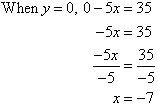y-intercept: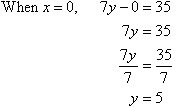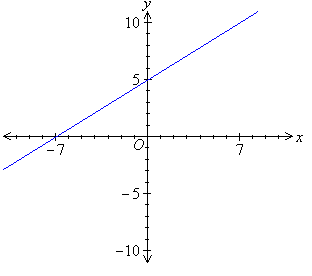###### Key Terms

Copyright © 2000-2019 mathsteacher.com Pty Ltd.  All rights reserved.
Australian Business Number 53 056 217 611

Copyright instructions for educational institutions

Please read the Terms and Conditions of Use of this Website and our Privacy and Other Policies.
If you experience difficulties when using this Website, tell us through the feedback form or by phoning the contact telephone number.